# General Procedures Sub Procedures Part II Function Procedures

• Slides: 10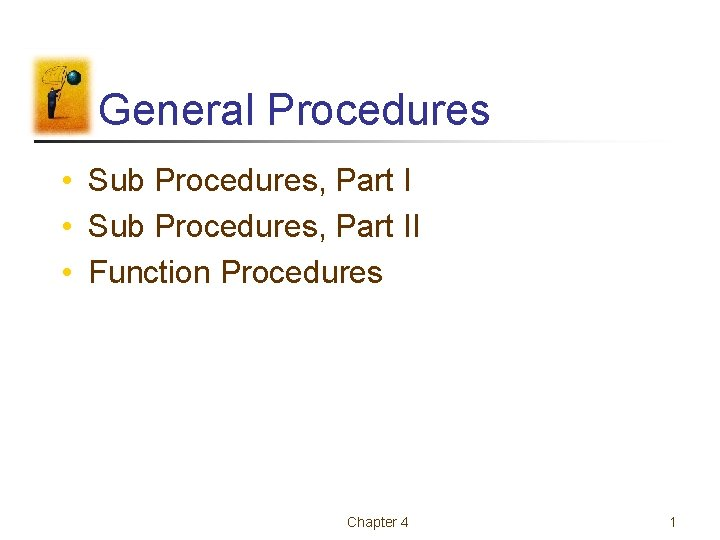General Procedures • Sub Procedures, Part II • Function Procedures Chapter 4 1Sub Procedures, Part I • Sub Procedures • Variables and Expressions as Arguments • Calling Other Sub Procedures Chapter 4 2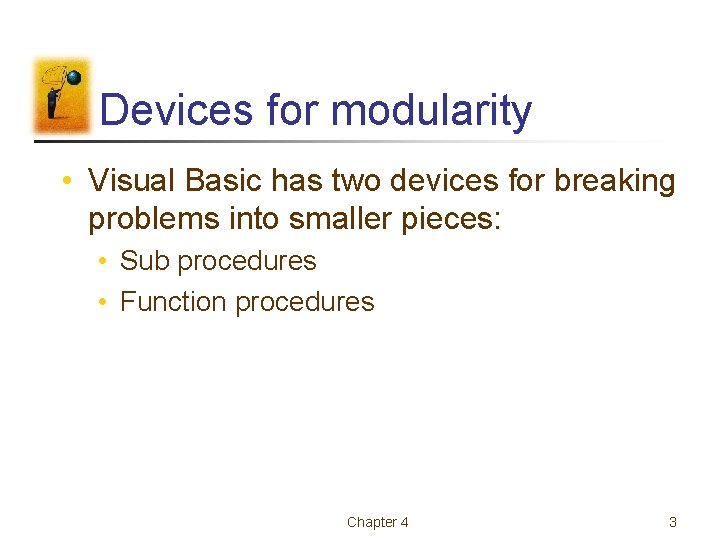Devices for modularity • Visual Basic has two devices for breaking problems into smaller pieces: • Sub procedures • Function procedures Chapter 4 3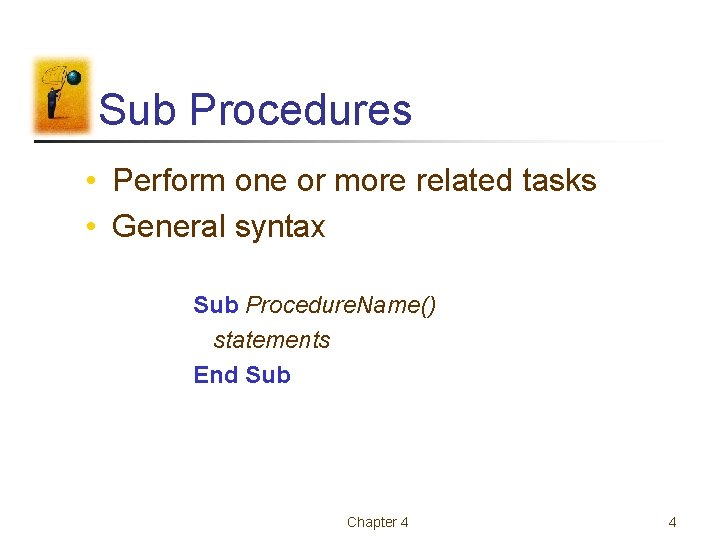Sub Procedures • Perform one or more related tasks • General syntax Sub Procedure. Name() statements End Sub Chapter 4 4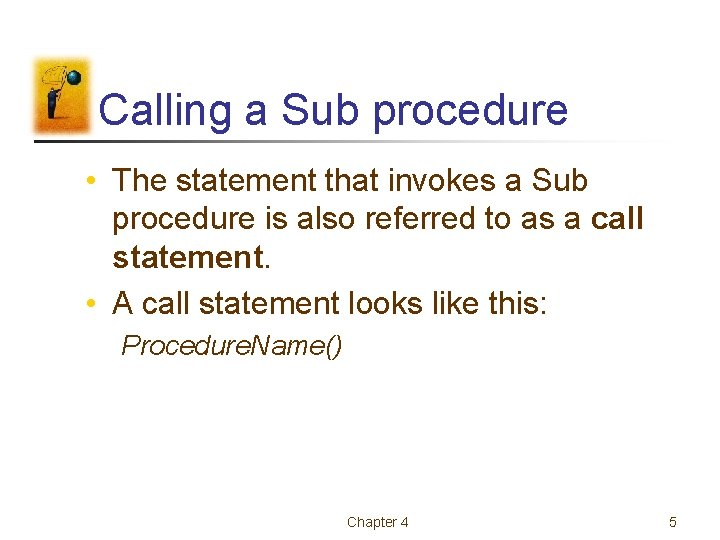Calling a Sub procedure • The statement that invokes a Sub procedure is also referred to as a call statement. • A call statement looks like this: Procedure. Name() Chapter 4 5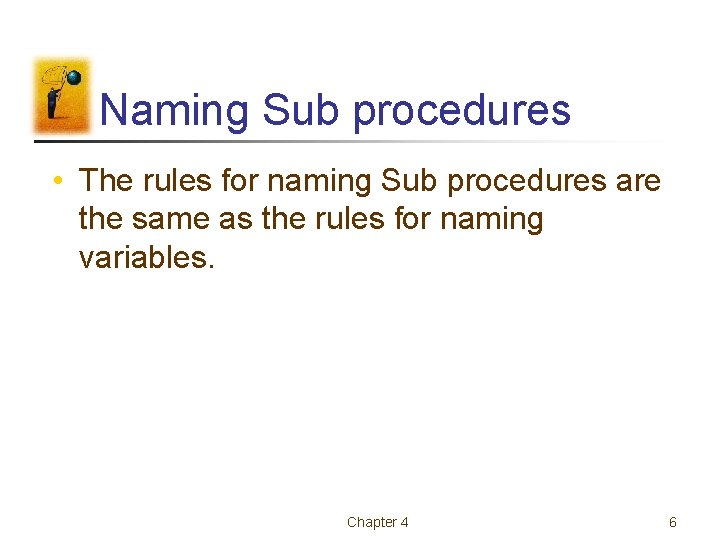Naming Sub procedures • The rules for naming Sub procedures are the same as the rules for naming variables. Chapter 4 6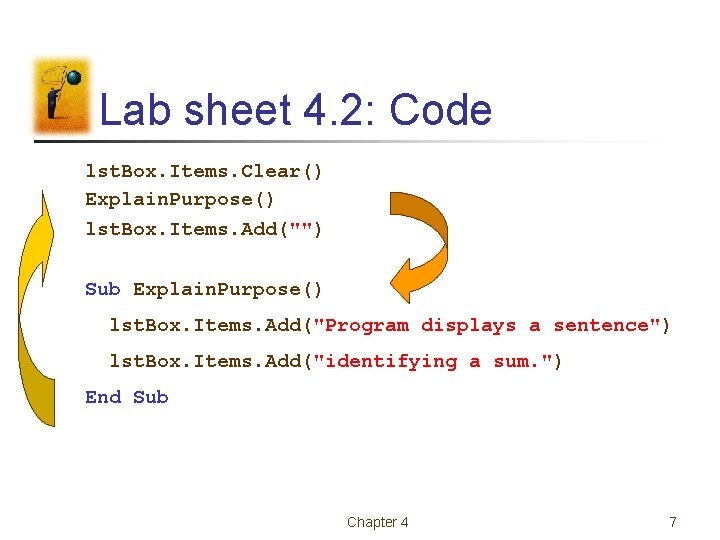Lab sheet 4. 2: Code lst. Box. Items. Clear() Explain. Purpose() lst. Box. Items. Add("") Sub Explain. Purpose() lst. Box. Items. Add("Program displays a sentence") lst. Box. Items. Add("identifying a sum. ") End Sub Chapter 4 7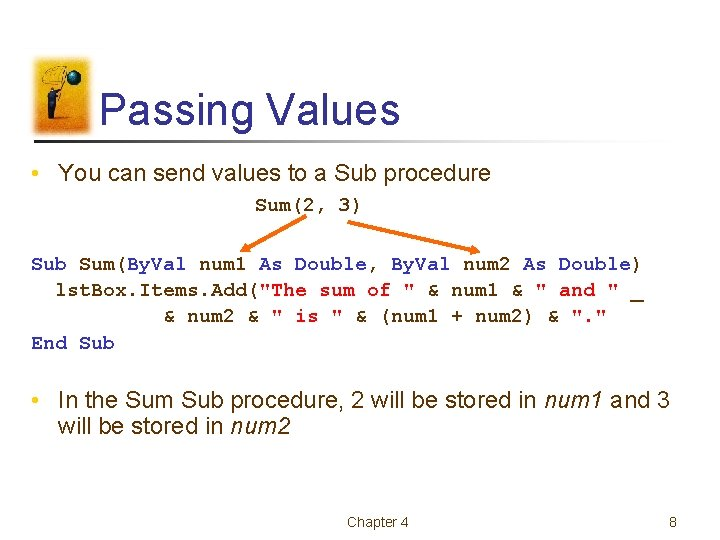Passing Values • You can send values to a Sub procedure Sum(2, 3) Sub Sum(By. Val num 1 As Double, By. Val num 2 As Double) lst. Box. Items. Add("The sum of " & num 1 & " and " _ & num 2 & " is " & (num 1 + num 2) & ". " End Sub • In the Sum Sub procedure, 2 will be stored in num 1 and 3 will be stored in num 2 Chapter 4 8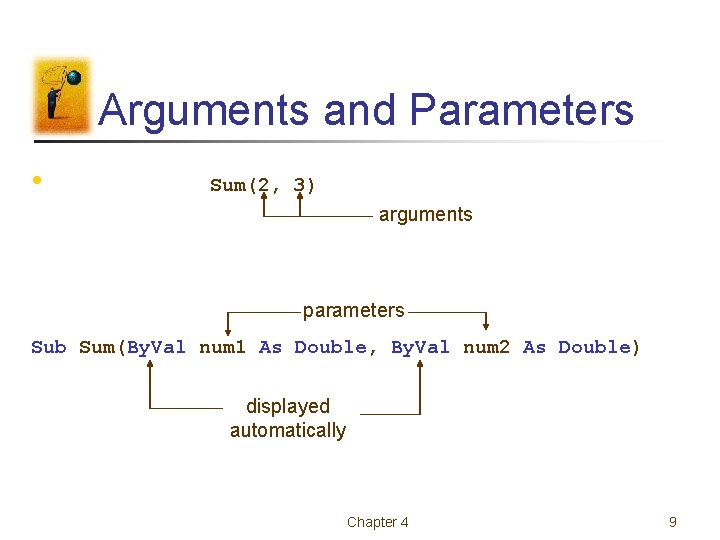Arguments and Parameters • Sum(2, 3) arguments parameters Sub Sum(By. Val num 1 As Double, By. Val num 2 As Double) displayed automatically Chapter 4 9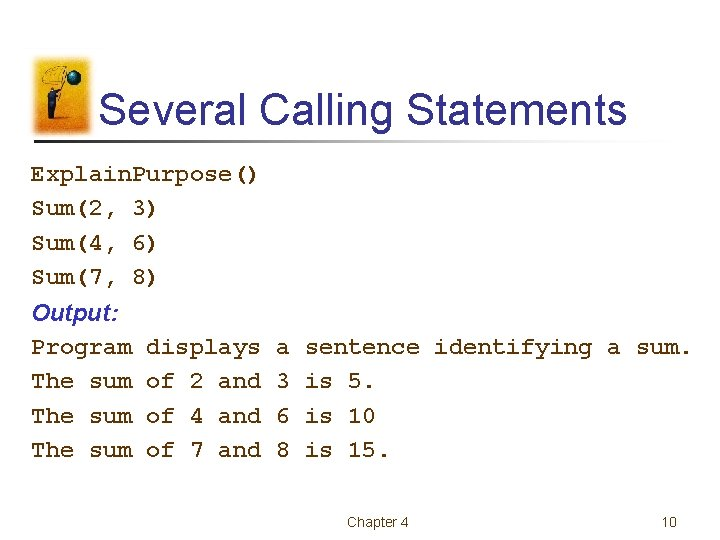Several Calling Statements Explain. Purpose() Sum(2, 3) Sum(4, 6) Sum(7, 8) Output: Program The sum displays of 2 and of 4 and of 7 and a 3 6 8 sentence identifying a sum. is 5. is 10 is 15. Chapter 4 10